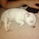948 views
Return the value of a simple moving average with a period within the range min to max such that the variance of the same period is the smallest available.

Since the smallest variance is often the one with the smallest period, a penalty setting is introduced, and allows the indicator to return moving averages values with higher periods more often, with higher penalty values returning moving averages values with higher periods.

Because variances with smaller periods are more reactive than ones with higher periods, it is common for the indicator to return the value of an SMA of a higher period during more volatile market, this can be seen on the image below:

here variances from period 10 to 15 are plotted, a blueish color represents a higher period, note how they are the smallest ones when fluctuations are more volatile.

Indicator with min = 50, max = 200 and penalty = 0.5

In blue the indicator with penalty = 0, in red with penalty = 1, with both min = 50 and max = 200.

On The Script

The script minimize Var(i)/p with i ∈ (min,max) and p = i^penalty, this is done by computing the variance for each period i and keeping the smallest one currently in the loop, if we get a variance value smaller than the previously one found we calculate the value of an SMA with period i, as such the script deal with brute force optimization.

For our use case it is not possible to use the built-in sma and variance functions within a loop, as such we use cumulative forms for both functions.
Patreon: https://www.patreon.com/alexgrover